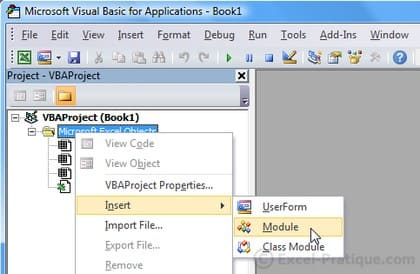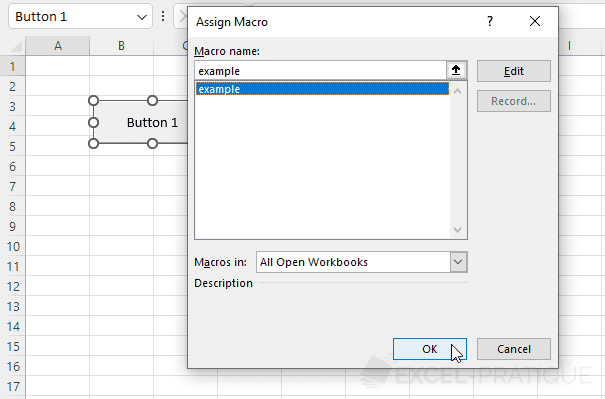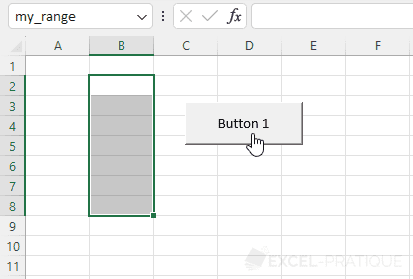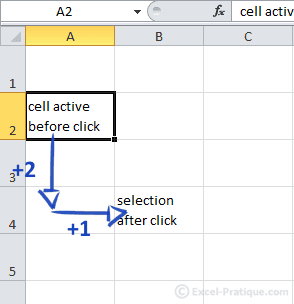VBA Course: Selections

We'll begin by creating a macro that selects the cell that we specifiy.

First open the editor and add a module:In the module, type "sub example" and press Enter.

You will notice that Excel has automatically filled in the end of this new procedure:

Sub example()

End Sub

Now create a formula button to which you will associate this macro (it is empty for now):Complete your macro with this code:

Sub example()
'Select cell A8
Range("A8").Select
End Sub

You can test this macro by clicking on your formula button, and you will see that cell A8 is now selected.

We will now edit the macro so that it selects cell A8 on the second worksheet:

Sub example()
'Activating of Sheet 2
Sheets("Sheet2").Activate
'Selecting of Cell A8
Range("A8").Select
End Sub

Excel will now activate Sheet 2 and then select cell A8.

Comments (text in green) will help you understand the macros in this course correctly.

Selecting different cells

Sub example()
'Selecting A8 and C5
Range("A8, C5").Select
End Sub

Selecting a range of cells

Sub example()
'Selecting cells A1 to A8
Range("A1:A8").Select
End Sub

Selecting a range of cells that has been renamed

Sub example()
'Selecting cells from the "my_range" range
Range("my_range").Select
End SubSelecting a cell by row and column number

Sub example()
'Selecting the cell in row 8 and column 1
Cells(8, 1).Select
End Sub

This method of selecting cells allows for more dynamic selections. It will be quite useful further along.

Here is a little example:

Sub example()
'Random selection of a cell from row 1 to 10 and column 1
Cells(Int(Rnd * 10) + 1, 1).Select
'Translation:
'Cells([random_number_between_1_and_10], 1).Select
End Sub

In this case, the row number is: Int(Rnd * 10) + 1, or in other words: a number between 1 and 10 (there's no reason you should learn this code at this point).

Moving a selection

Sub example()
'Selecting a cell (described in relation to the cell that is currently active)
ActiveCell.Offset(2, 1).Select
End Sub

Moving the selection box two rows down and one column to the right:Selecting rows

It is possible to select entire rows using the Range or Rows commands (the Rows command is of course specific to rows).

Sub example()
'Selecting rows 2 to 6
Range("2:6").Select
End Sub
Sub example()
'Selecting rows 2 to 6
Rows("2:6").Select
End Sub

Selecting columns

As with rows, it is possible to select entire columns using the Range or Columns commands (the Columns command is of course specific to columns).

Sub example()
'Selecting columns B to G
Range("B:G").Select
End Sub
Sub example()
'Selecting columns B to G
Columns("B:G").Select
End Sub